# Altitude of a rocket- mass vs initial speedPage 2

#### WATCH ALL SLIDES

Left

Right

#4

Mass = 10

Speed = 10

Mass = 100

Speed = 100

Correct!

Slide 18#5

Slide 19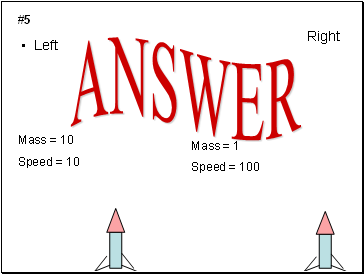Left

Right

#5

Mass = 10

Speed = 10

Mass = 1

Speed = 100

Slide 20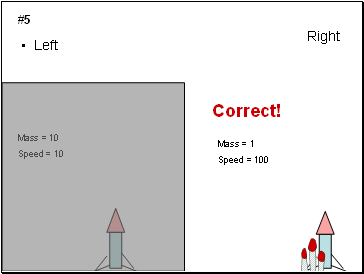Left

Right

#5

Mass = 10

Speed = 10

Mass = 1

Speed = 100

Correct!

Slide 21#6

Slide 22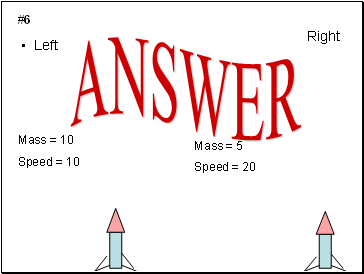Left

Right

#6

Mass = 10

Speed = 10

Mass = 5

Speed = 20

Slide 23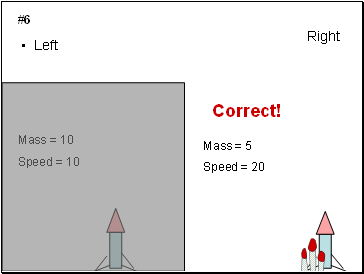Left

Right

#6

Mass = 10

Speed = 10

Mass = 5

Speed = 20

Correct!

Slide 24#7

Slide 25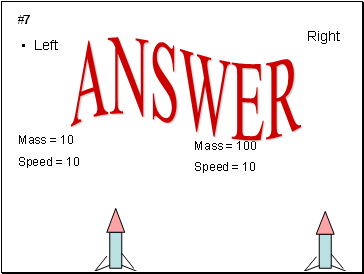Left

Right

#7

Mass = 10

Speed = 10

Mass = 100

Speed = 10

Slide 26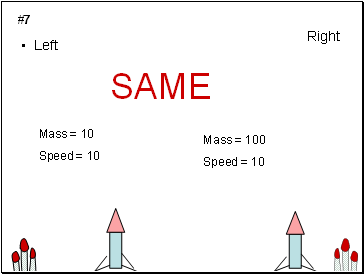Left

Right

#7

Mass = 10

Speed = 10

Mass = 100

Speed = 10

SAME

Slide 27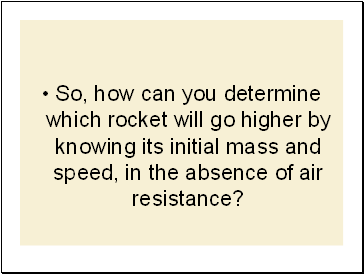So, how can you determine which rocket will go higher by knowing its initial mass and speed, in the absence of air resistance?

Slide 28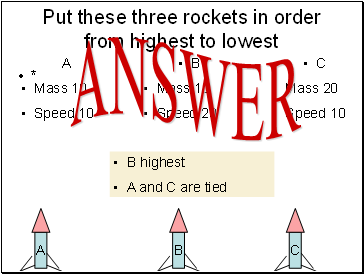Put these three rockets in order from highest to lowest

*

A

Mass 10

Speed 10

B

Mass 10

Speed 20

C

Mass 20

Speed 10

B highest

A and C are tied

Slide 29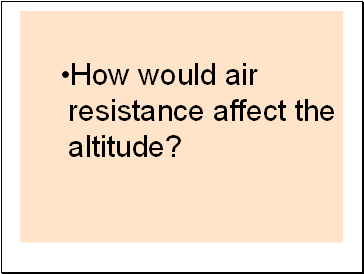How would air resistance affect the altitude?

Go to page:
1  2